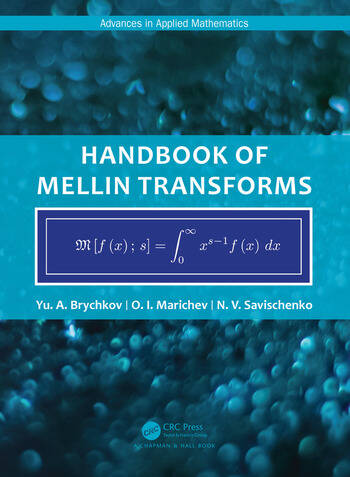# Handbook of Mellin Transforms

## 1st Edition

Yu. A. Brychkov, O. I. Marichev, N. V. Savischenko

Chapman and Hall/CRC
Published October 2, 2018
Reference - 587 Pages
ISBN 9781138353350 - CAT# K395513

was \$299.00

USD\$239.20

SAVE ~\$59.80

FREE Standard Shipping!

## Preview

### Summary

The Mellin transformation is widely used in various problems of pure and applied mathematics, in particular, in the theory of differential and integral equations and the theory of Dirichlet series. It is found in extensive applications in mathematical physics, number theory, mathematical statistics, theory of asymptotic expansions, and especially, in the theory of special functions and integral transformations. It is essentially used in algorithms of integration in computer algebra systems.

Since the majority of integrals encountered in applications can be reduced to the form of the corresponding Mellin transforms with specific parameters, this handbook can also be used for definite and indefinite integrals. By changes in variables, the Mellin transform can be turned into the Fourier and Laplace transforms.

The appendices contain formulas of connection with other integral transformations, and an algorithm for determining regions of convergence of integrals.

The Handbook of Mellin Transforms will be of interest and useful to all researchers and engineers who use mathematical methods. It will become the main source of formulas of Mellin transforms, as well as indefinite and definite integrals.